# Right triangle - 9th grade (14y) - examples - page 20

1. The sides 2The sides of a trapezoid are in the ratio 2:5:8:5. The trapezoid’s area is 245. Find the height and the perimeter of the trapezoid.
2. Diamond diagonalsFind the diamond diagonal's lengths if the area is 156 cm2 and side is 13 cm long.
3. Right triangleCalculate the length of the remaining two sides and the angles in the rectangular triangle ABC if a = 10 cm, angle alpha = 18°40'.
4. Body diagonalCalculate the length of the body diagonal of the 6cm cube.
5. Cube diagonalsThe cube has a wall area of 81 cm square. Calculate the length of its edge, wall, and body diagonal.
6. Find radiusFind radius of circle using pythagorean theorem where a=9, b=r, c= 6+r
7. Construct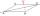Construct a rhombus ABCD, if the size of the diagonal AC is 6 cm and diagonal BD 8 cm long.
8. Cone 15The radius of the base of a right circular cone is 14 inches and it's height 18 inches. What is the slant height?
9. Cuboidal room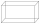Length of cuboidal room is 2m breadth of cuboidal room is 3m and height is 6m find the length of the longest rod that can be fitted in the room
10. The pole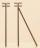The telegraph pole is supported by a 4 m bullet at 3/4 of its height, the end of which is at a distance 2.5 m from the pole post. Calculate the height of the telegraph pole.
11. Tetrahedron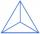What is the angle of the sides from the base of a three-sided pyramid where the sides are identical?
12. Pendulum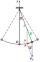Calculate the length of the pendulum that is 2 cm lower in the lowest position than in the highest position. The length of the circular arc to be described when moving is 20cm.
13. Quadrangular pyramidThe regular quadrangular pyramid has a base length of 6 cm and a side edge length of 9 centimeters. Calculate its volume and surface area.
14. Prism 4 sidesThe prism has a square base with a side length of 3 cm. The diagonal of the sidewall of the prism/BG/is 5 cm. Calculate the surface of this prism in cm square and the volume in liters
15. Body diagonal - cubeCalculate the surface and cube volume with body diagonal 15 cm long.
16. Prism diagonalThe body diagonal of a regular square prism has an angle of 60 degrees with the base, the edge length is 10 cm. What is the volume of the prism?
17. Vertices of a right triangleShow that the points D(2,1), E(4,0), F(5,7) are vertices of a right triangle.
18. Cross roadFrom the junction of two streets that are perpendicular to each other, two cyclists (each on another street) walked out. One ran 18 km/h and the second 24 km/h. How are they away from a) 6 minutes, b) 15 minutes?
19. Body diagonalCalculate the cube volume, whose body diagonal size is 75 dm. Draw a picture and highlight the body diagonal.
20. Slant heightThe slant height of cone is 5cm and the radius of its base is 3cm, find the volume of the cone

Do you have an interesting mathematical example that you can't solve it? Enter it, and we can try to solve it.

To this e-mail address, we will reply solution; solved examples are also published here. Please enter e-mail correctly and check whether you don't have a full mailbox.

See also our right triangle calculator.